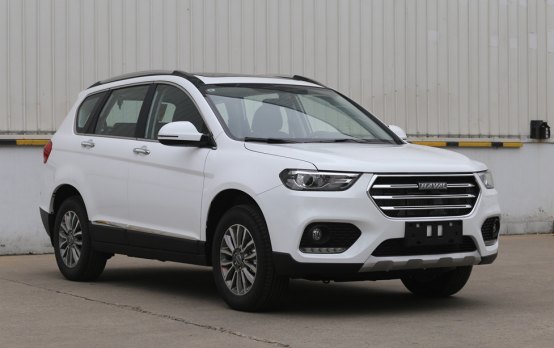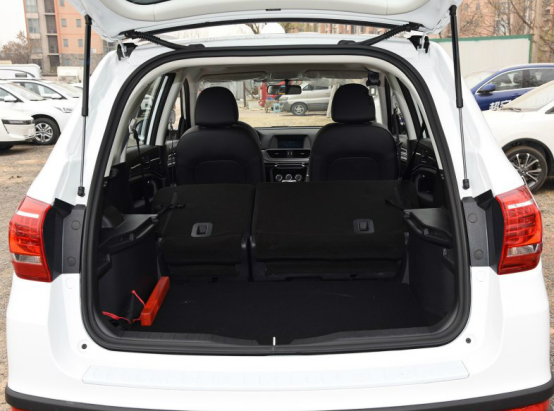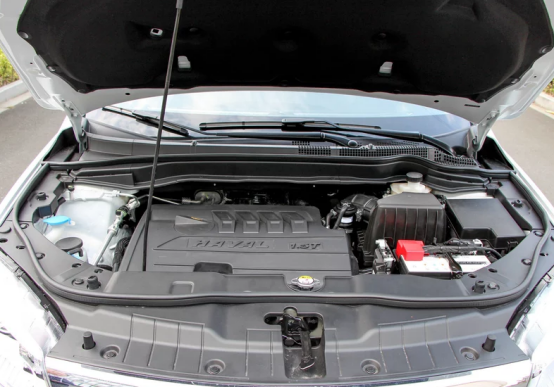## 哈弗H6在手 说走就走—自驾游最佳拍档

2019年07月08日 10:46 来源：车行天下 超过：8183次关注

2019年7月初，地点北京，天气酷暑难耐。

“太热了，无空调不成活啊”，这是众多在北京三伏天儿里上班人的感叹。

从六月底开始，北京的温度就高居不下，远离空调都是一种煎熬，但一直待在室内又很无聊。这时，我的逃离市区避暑的提议得到了小伙伴的响应，计划双休日进行避暑逃离之旅。面对五花八门跟团游极高的入坑率和搭车前往中途转车极高的麻烦率，我和小伙伴都倾向自驾游。一般出发自驾游，总会有亲友担心嘱咐“注意安全”，确实，漫漫旅途安全是第一要素，车辆的选择至关重要。这次我和小伙伴选择驾驶哈弗H6运动版，从市区出发前往古北水镇，感受清凉舒适的自驾之旅。但是，自驾游不同于日常跑高速，存在许多不确定因素，在出发之前需要做好充足的准备。除了预订酒店等还需要带上身份证、行驶证、驾照等各种证件、现金、适时衣物、保温水壶、餐具、雨伞、手套、适合驾驶的软底鞋、雨具，还有各种药品、食品等。要想有一个愉快的自驾游，这些准备都必不可少。可能会有疑问这么多东西放私家车上，路途坐着能舒服吗?不用担心，哈弗H6运动版空间惊人，其长*宽*高达到了4649x1852x1710(mm)，更有808L-2010L的行李箱空间，这样优秀的空间表现不仅能把必需品“一网打尽”，还能带上各式各样的露营烧烤设备等，为旅行增添乐趣。我和小伙伴把乱七八糟的行李放上去后，后排完全空荡荡的，一点都不挤占乘坐空间，即使一家出行也够用。准备充足后，周六一大早就驾驶哈弗H6运动版前往古北水镇。古北水镇位于密云区，从北京市内出发沿京承高速行驶，在司马台出口下高速后沿马北路行驶，路程共120公理，路上行驶过高速、国道还有乡间小路等。得益于哈弗H6运动版靠谱的动力性能，开车一路狂奔，颇有一种“久在樊笼里，复得返自然”的自由和畅快感。当然，这就不得不提汽车的1.5T高效节能涡轮增压发动机和格特拉克7速DCT湿式双离合变速器了，发动机最大功率110kW，最大扭矩210N•m，动力完全充足，而且换挡平顺，衔接顺畅，我和小伙伴一路上丝毫不担心路不好走导致车辆行驶不畅。从市区驱车到古北水镇，路途不算遥远，但也需要1个半小时。在夏季高温情况下，对于胎压是个考验，但是哈弗H6运动版的智能胎压监测系统就能很好保驾护航，可以对轮胎气压和温度进行实时自动监测，如果胎压过高会及时报警，驾驶时心里确实会多一份安全感。很多时候自驾游还会碰到这样的情况：半路上，在驾驶座的司机都快冒烟了，幸好有空调才捡回一条命，这时往往副驾驶会跳出来说：“有点儿凉，把空调调高点吧。”就空调温度问题，司机和乘客有时都能打起来。但是我和小伙伴一路上都很和谐，并不是因为两个人体感温度一致，而是有哈弗H6运动版的双温区自动空调，能让每个人都能享受最舒适的温度，收获极佳的旅途体验。

最终，我们赶在中午前到达了古北水镇，一路上没碰到大的意外。哈弗H6运动版丰富的安全装置确实帮忙不少，给我这个开车新手增添了不少信心，一路上开车操作都能抵得上老司机了。一个半小时的车程也让我和小伙伴体验到了哈弗H6运动版的座椅舒适度，到达时完全感觉不到腰酸腿疼，都精神饱满，到达即浪。这是一次奇妙的自驾游体验，要是也想和我一样有一次极佳的周末避暑游，找个哈弗H6运动版完全ok。更重要的是，在国六排放标准正式实施之际，哈弗H6运动版国六车型也正式开启预售，动力不打折，配置更丰富。在7月1日-8月31日，哈弗即日开启了“国六来袭 钜惠升级 H6运动版6.6抵666元 享至高2.8万综合钜惠”活动。哈弗H6运动版至高综合优惠28000元，而且消费者支付6.6元即可享受到666元的购车金，同时哈弗还邀您参加“哈弗邀您团战一夏”活动，可以以更低的价格享受维修保养服务、夏日空调养护服务等，还可以用会员积分兑换工时券，而且在哈弗智家APP论坛发帖，有机会获得500积分。赶紧去长城汽车首家品牌体验中心瞅瞅呐，多样化的服务绝对给你最佳体验。

#### 相关文章

0-500 字已有评论 0条 查看评论>>

### 热门标签

﻿
• 快速找车
• 选择品牌
• 选择品牌
• A  奥迪
• A  阿斯顿·马丁
• A  阿尔法·罗密欧
• B  宝沃
• B  布加迪
• B  巴博斯
• B  保时捷
• B  宾利
• B  奔驰
• B  宝马
• B  本田
• B  别克
• B  标致
• B  比亚迪
• B  宝骏
• B  北汽制造
• B  北汽新能源
• B  北汽幻速
• B  北汽威旺
• B  北京汽车
• B  奔腾
• B  北汽绅宝
• C  长安
• C  长安商用
• C  长城
• C  昌河
• D  大众
• D  道奇
• D  DS
• D  东南
• D  东风风神
• D  东风风行
• D  东风小康
• D  东风风度
• D  东风
• F  福特
• F  丰田
• F  菲亚特
• F  法拉利
• F  福田
• F  福迪
• F  福汽启腾
• G  观致
• G  广汽传祺
• G  广汽吉奥
• G  GMC
• H  红旗
• H  汉腾汽车
• H  哈弗
• H  哈飞
• H  海格
• H  海马
• H  华颂
• H  黄海
• H  华泰
• H  恒天
• J  吉利汽车
• J  捷豹
• J  Jeep
• J  江淮
• J  江铃
• J  金杯
• J  九龙
• J  金旅
• K  凯翼
• K  凯迪拉克
• K  克莱斯勒
• K  科尼塞克
• K  卡威
• K  开瑞
• L  路虎
• L  林肯
• L  劳斯莱斯
• L  兰博基尼
• L  雷克萨斯
• L  铃木
• L  雷诺
• L  理念
• L  力帆
• L  莲花汽车
• L  猎豹
• L  路特斯
• L  陆风
• M  马自达
• M  MG
• M  MINI
• M  玛莎拉蒂
• M  摩根
• M  迈凯轮
• N  纳智捷
• O  欧宝
• O  讴歌
• O  欧朗
• Q  奇瑞
• Q  起亚
• Q  启辰
• R  日产
• R  荣威
• R  瑞麒
• S  三菱
• S  斯威汽车
• S  萨博
• S  smart
• S  斯柯达
• S  斯巴鲁
• S  思铭
• S  双龙
• S  上汽大通
• S  双环
• T  特斯拉
• T  腾势
• W  沃尔沃
• W  五菱汽车
• W  五十铃
• W  威兹曼
• W  威麟
• X  现代
• X  雪佛兰
• X  雪铁龙
• X  西雅特
• Y  一汽
• Y  英菲尼迪
• Y  英致
• Y  依维柯
• Y  野马汽车
• Y  永源
• Z  众泰
• Z  中华
• Z  中兴
• Z  知豆
• 选择车系
• 选择车系
• 车型对比
• 选择品牌
• 选择品牌
• A  奥迪
• A  阿斯顿·马丁
• A  阿尔法·罗密欧
• B  宝沃
• B  布加迪
• B  巴博斯
• B  保时捷
• B  宾利
• B  奔驰
• B  宝马
• B  本田
• B  别克
• B  标致
• B  比亚迪
• B  宝骏
• B  北汽制造
• B  北汽新能源
• B  北汽幻速
• B  北汽威旺
• B  北京汽车
• B  奔腾
• B  北汽绅宝
• C  长安
• C  长安商用
• C  长城
• C  昌河
• D  大众
• D  道奇
• D  DS
• D  东南
• D  东风风神
• D  东风风行
• D  东风小康
• D  东风风度
• D  东风
• F  福特
• F  丰田
• F  菲亚特
• F  法拉利
• F  福田
• F  福迪
• F  福汽启腾
• G  观致
• G  广汽传祺
• G  广汽吉奥
• G  GMC
• H  红旗
• H  汉腾汽车
• H  哈弗
• H  哈飞
• H  海格
• H  海马
• H  华颂
• H  黄海
• H  华泰
• H  恒天
• J  吉利汽车
• J  捷豹
• J  Jeep
• J  江淮
• J  江铃
• J  金杯
• J  九龙
• J  金旅
• K  凯翼
• K  凯迪拉克
• K  克莱斯勒
• K  科尼塞克
• K  卡威
• K  开瑞
• L  路虎
• L  林肯
• L  劳斯莱斯
• L  兰博基尼
• L  雷克萨斯
• L  铃木
• L  雷诺
• L  理念
• L  力帆
• L  莲花汽车
• L  猎豹
• L  路特斯
• L  陆风
• M  马自达
• M  MG
• M  MINI
• M  玛莎拉蒂
• M  摩根
• M  迈凯轮
• N  纳智捷
• O  欧宝
• O  讴歌
• O  欧朗
• Q  奇瑞
• Q  起亚
• Q  启辰
• R  日产
• R  荣威
• R  瑞麒
• S  三菱
• S  斯威汽车
• S  萨博
• S  smart
• S  斯柯达
• S  斯巴鲁
• S  思铭
• S  双龙
• S  上汽大通
• S  双环
• T  特斯拉
• T  腾势
• W  沃尔沃
• W  五菱汽车
• W  五十铃
• W  威兹曼
• W  威麟
• X  现代
• X  雪佛兰
• X  雪铁龙
• X  西雅特
• Y  一汽
• Y  英菲尼迪
• Y  英致
• Y  依维柯
• Y  野马汽车
• Y  永源
• Z  众泰
• Z  中华
• Z  中兴
• Z  知豆
• 选择车系
• 选择车系
• 选择车型
• 选择车型
• 意见反馈